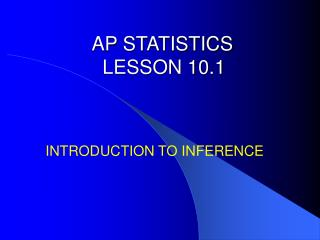DownloadDownload PresentationAP STATISTICS LESSON 10.1

# AP STATISTICS LESSON 10.1

Télécharger la présentation## AP STATISTICS LESSON 10.1

- - - - - - - - - - - - - - - - - - - - - - - - - - - E N D - - - - - - - - - - - - - - - - - - - - - - - - - - -
##### Presentation Transcript

1. AP STATISTICSLESSON 10.1 INTRODUCTION TO INFERENCE

2. ESSENTIAL QUESTION: What is a confidence interval and how are a they used to make inferences? Objectives: • To find confidence intervals. • To interpret the meaning of confidence intervals.

3. Introduction Often we are not content with information about the sample. We want to infer from the sample data some conclusion about a wider population that the sample represents.

4. Statistical Inference Statistical inference provides methods for drawing conclusions about a population from sample data. We use probability to express the strength of our conclusions. Probability allows us to take chance variation into account and so to correct our judgment by calculations.

5. Example 10.1 page 536Draft Lotteries and Drug Studies In the Vietnam War years, a lottery determined the order in which men were drafted for army service. The lottery assigned draft numbers by choosing birth dates in random order. We expect a correlation of about zero. The actual correlation between birth date and draft number in the first draft lottery was r = - .226 That is men born later in the year tended to get lower draft numbers. The probability of the correlation being that far from 0 by chance is 0.001 in a truly random lottery.

6. Estimating With Confidence A computer will do the arithmetic, but you must still exercise judgment based on understanding. The methods of formal inference require the long-run regular behavior that probability describes. Inference is most reliable when the data are produced by a properly randomized design. When you use statistical inference you are acting as if the data are random sample or come from a randomized experiment. If this is not true, your conclusions may be open to challenge.

7. Example 10.1 (continued…) Suppose that we know that the standard deviation of SAT math is σ = 100 σ/√n = 100/√500 = 4.5 x = 463 Inference about the unknown μ starts from this sample distribution. Figure 10.1 shows different SRS of 500 California seniors and a graph of their distribution.

8. Example 10.2 page 536SAT Math Scores in California In 2000, 1,260,278 college bound seniors took the SAT. Their mean SAT math score was 514 with a standard deviation of 113. For the SAT verbal, the mean was 505 with a standard deviation of 111. Suppose you want to estimate the mean SAT math score for the more than 350,000 high school seniors in California. Only 49% of the California students take the SAT. These self-selected seniors are planning to attend college and so are not representative of all college seniors. You give the test to an SRS of 500 high school seniors and determine mean x = 461. What can you say about the mean score μ in the population of all 350,000 seniors?

9. Essential Facts About Sampling Distribution of x. • The central limit theorem tells us that the mean x of 500 scores has a distribution that is close to normal. • The mean of this normal sampling distribution is the same as the unknown mean μ of the entire population. • The standard deviation of x for an SRS of 500 students is σ/ √ 500, where σ is the standard deviation of individual SAT math scores among all California high school seniors.

10. Statistical Confidence • The 68-95-99.7 rule says that in 95% of all samples, the mean score x for the sample will be within two standard deviations of the population mean score μ. So the mean x of 500 SAT math scores will be within 9 points of μ in 95% of all samples. • Whenever x is within 9 points of the unknown μ, μ is within 9 points of the observed x. This happens in 95% of all samples. • So in 95% of all samples, the unknown μ lies between x – 9 and x + 9.

11. Figures 10.2 and 10.3 Page 539

12. Example 10.3 page 54095% Confidence Our sample of 500 California seniors gave X = 461. We say that we are 95% confident that the unknown SAT math sore for all California high school seniors lies between 452 and 470. Be sure you understand the grounds for our confidence. There are only two possibilities: • The interval between 452 and 470 contains the true μ. • Our SRS was one of the few samples for which x is not within 9 points of the true μ. Only 5% of all samples give such inaccurate results.

13. Example 10.3 (continued…) Margin of Error - The margin of error ± 9 shows how accurate we believe our guess is, based on the variability of the estimate. This is a 95% confidence interval because it catches the unknown μ in 95% of all possible samples.

14. Confidence interval A level C confidence interval for a parameter has two parts: 1. An interval calculated from the data, usually of the form estimate ± margin of error 2. A confidence level C, which gives the probability that the interval will capture the true parameter value in repeated samples. (Use decimal form for %.)

15. Figure 10.4 Page 541 The graph shows that only one interval does not contain parameters mean.